×#### Thank you for registering.

One of our academic counsellors will contact you within 1 working day.

Click to Chat

1800-1023-196

+91-120-4616500

CART 0

• 0

MY CART (5)

Use Coupon: CART20 and get 20% off on all online Study Material

ITEM
DETAILS
MRP
DISCOUNT
FINAL PRICE
Total Price: Rs.

There are no items in this cart.
Continue Shopping• Complete JEE Main/Advanced Course and Test Series
• OFFERED PRICE: Rs. 15,900
• View Details

```Chapter 8: Lines And Angles Exercise – 8.2

Question: 1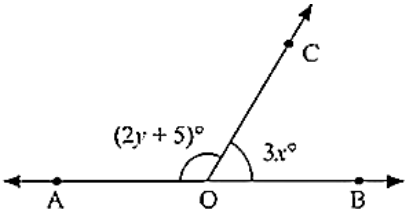In the below Fig. OA and OB are opposite rays:

(i) If x = 25, what is the value of y?

(ii) If y = 35, what is the value of x?

Solution:

(i) Given that,

x = 25

Since ∠AOC and ∠BOC form a linear pair

∠AOC + ∠BOC = 180°

Given that ∠AOC = 2y + 5 and ∠BOC = 3x

∠AOC + ∠BOC = 180°

(2y + 5) + 3x = 180

(2y +5) + 3(25) = 180

2y + 5 + 75 = 180

2y + 80 = 180

2y = 180 - 80 = 100

y = 100/2 = 50

y = 50

(ii) Given that,

y = 35

∠AOC + ∠BOC = 180°

(2y + 5) + 3x = 180

(2(35) + 5) + 3x = 180

(70 + 5) + 3x = 180

3x = 180 - 75

3x = 105

x = 35

Question: 2

In the below figure, write all pairs of adjacent angles and all the linear pairs.Solution:

(i) ∠AOC, ∠COB

(ii) ∠AOD ∠BOD

(i) ∠AOD, ∠COD

(i) ∠BOC, ∠COD

Linear pairs: ∠AOD, ∠BOD, ∠AOC, ∠BOC

Question: 3

In the given below figure, find x. Further find ∠COD, ∠AOD, ∠BOC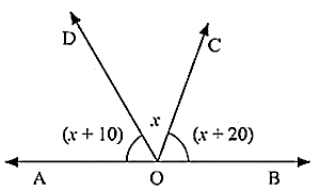Solution:

Since ∠AOD and ∠BOD form a line pair,

∠AOD + ∠BOD = 180°

∠AOD + ∠BOC + ∠COD = 180°

Given that,

∠AOD = (x + 10)°, ∠COD = x°, ∠BOC = (x + 20)°

(x + 10) + x + (x + 20) = 180

3x + 30 = 180

3x = 180 - 30

3x = 150/3

x = 50

Therefore, ∠AOD = (x + 10)

= 50 + 10 = 60

∠COD = x = 50°

∠COD = (x + 20)

= 50 + 20 = 70

∠AOD = 60°∠COD = 50°∠BOC = 70°

Question: 4

In the given below figure rays OA, OB, OC, OP and OE have the common end point O. Show that ∠AOB + ∠BOC + ∠COD + ∠DOE + ∠EOA = 360°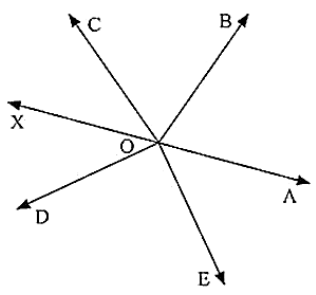Solution:

Given that OA, OB, OD and OE have the common end point O.

A ray opposite to OA is drawn

Since ∠AOB, ∠BOF are linear pairs,

∠AOB + ∠BOF = 180°

∠AOB + ∠BOC + ∠COF = 180°  .... (1)

Also,

∠AOE and ∠EOF are linear pairs

∠AOE + ∠EOF = 180°

∠AOE + ∠DOF + ∠DOE = 180°  .... (2)

By adding (1) and (2) equations we get

∠AOB + ∠BOC + ∠COF + ∠AOE + ∠DOF + ∠DOE = 180°

∠AOB + ∠BOC + ∠COD + ∠DOE + ∠EOA = 180°

Hence proved.

Question: 5

In the below figure, ∠AOC and ∠BOC form a linear pair. If a - 2b = 30°, find a and b?Solution:

Given that,

∠AOC and ∠BOC form a linear pair

If a - b = 30

∠AOC = a°, ∠BOC = b°

Therefore, a + b = 180 ... (1)

Given a - 2b = 30 ... (2)

By subtracting (1) and (2)

a + b - a + 2b = 180 - 30

3b = 150

b = 150/3

b = 50

Since a - 2b = 30

a - 2(50) = 30

a = 30 + 100

a = 130

Hence, the values of a and b are 130° and 50° respectively.

Question: 6

How many pairs of adjacent angles are formed when two lines intersect at a point?

Solution:

Four pairs of adjacent angles will be formed when two lines intersect at a point.

Considering two lines AB and CD intersecting at O

The 4 pairs are:

(∠AOD, ∠DOB), (∠DOB, ∠BOC), (∠COA, ∠AOD) and (∠BOC, ∠COA)

Hence, 4 pairs of adjacent angles are formed when two lines intersect at a point.

Question: 7

How many pairs of adjacent angles, in all, can you name in the figure below?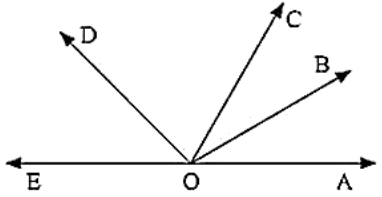Solution:

∠EOC, ∠DOC

∠EOD, ∠DOB

∠DOC, ∠COB

∠EOD, ∠DOA

∠DOC, ∠COA

∠BOC, ∠BOA

∠BOA, ∠BOD

∠BOA, ∠BOE

∠EOC, ∠COA

∠EOC, ∠COB

Hence, 10 pair of adjacent angles.

Question: 8

In the below figure, find value of x?Solution:

Since the sum of all the angles round a point is equal to 360°

3x + 3x + 150 + x = 360

7x = 360 - 150

7x = 210

x = 210/7

x = 30

Value of x is 30°

Question: 9

In the below figure, AOC is a line, find x.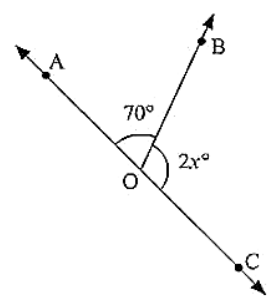Solution:

Since ∠AOB and ∠BOC are linear pairs,

∠AOB + ∠BOC = 180°

70 + 2x = 180

2x = 180 - 70

2x = 110

x = 110/2

x = 55

Hence, the value of x is 55°

Question: 10

In the below figure, POS is a line, Find x?Solution:

Since ∠POQ and ∠QOS are linear pairs

∠POQ + ∠QOS = 180°

∠POQ + ∠QOR + ∠SOR = 180°

60 + 4x + 40 = 180

4x = 180 - 100

4x = 80

x = 20

Hence, Value of x = 20

Question: 11

In the below figure, ACB is a line such that ∠DCA = 5x and ∠DCB = 4x. Find the value of x?Solution:

Here, ∠ACD + ∠BCD = 180°

[Since they are linear pairs]

∠DCA = 5x and ∠DCB = 4x

5x + 4x = 180

9x = 180

x = 20

Hence, the value of x is 20°

Question: 12

In the given figure, Given ∠POR = 3x and ∠QOR = 2x + 10, Find the value of x for which POQ will be a line?Solution:

For the case that POR is a line

∠POR and ∠QOR are linear parts

∠POR + ∠QOR = 180°

Also, given that,

∠POR = 3x and ∠QOR = 2x + 10

2x + 10 + 3x = 180

5x + 10 = 180

5x = 180 – 10

5x = 170

x = 34

Hence the value of x is 34°

Question: 13

In Fig: a is greater than b by one third of a right angle. Find the value of a and b?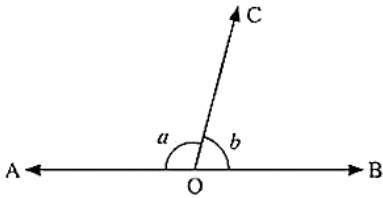Solution:

Since a and b are linear

a + b = 180

a = 180 – b  ... (1)

From given data, a is greater than b by one third of a right angle

a = b + 90/3

a = b + 30

a – b = 30 ... (2)

Equating (1) and (2)

180 – b = b + 30

180 – 30 = 2b

b = 150 / 2

b = 75

From (1)

a = 180 – b

a = 180 – 75

a = 105

Hence the values of a and b are 105° and 75° respectively.

Question: 14

What value of y would make AOB a line in the below figure, If ∠AOB = 4y and ∠BOC = (6y + 30)?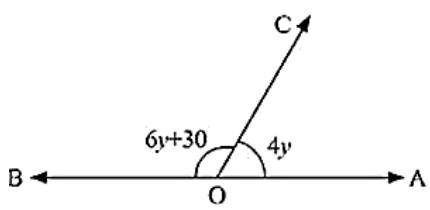Solution:

Since, ∠AOC and ∠BOC are linear pairs

∠AOC + ∠BOC = 180°

6y + 30 + 4y = 180

10y + 30 = 180

10y = 180 - 30

10y = 150

y = 150/10

y = 15

Hence value of y that will make AOB a line is 15°

Question: 15

If the figure below forms a linear pair,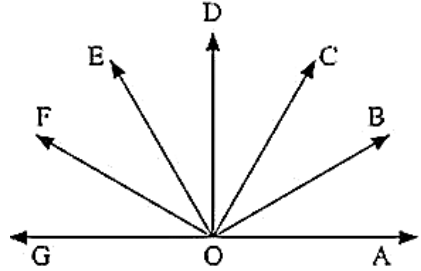∠EOB = ∠FOC = 90 and ∠DOC = ∠FOG = ∠AOB = 30

Find the measure of ∠FOE, ∠COB and ∠DOE

Name all the right angles

Name three pairs of adjacent complementary angles

Name three pairs of adjacent supplementary angles

Name three pairs of adjacent angles

Solution:

(i) ∠FOE = x, ∠DOE = y and ∠BOC = z

Since ∠AOF, ∠FOG is a linear pair

∠AOF + 30 = 180

∠AOF = 180 - 30

∠AOF = 150

∠AOB + ∠BOC + ∠COD + ∠DOE + ∠EOF = 150

30 + z + 30 + y + x = 150

x + y + z =150 - 30 - 30

x + y + z = 90  .... (1)

∠FOC = 90°

∠FOE + ∠EOD + ∠DOC = 90°

x + y + 30 = 90

x + y = 90 - 30

x + y =60 ... (2)

Substituting (2) in (1)

x + y + z = 90

60 + z = 90

z = 90 - 60 = 30

Given BOE = 90

∠BOC + ∠COD + ∠DOE = 90°

30 + 30 + DOE = 90

DOE = 90 - 60 = 30

DOE = x = 30

We also know that,

x + y = 60

y = 60 - x

y = 60 - 30

y = 30

Thus we have ∠FOE = 30, ∠COB = 30 and ∠DOE = 30

(ii) Right angles are ∠DOG, ∠COF, ∠BOF, ∠AOD

(iii) Adjacent complementary angles are (∠AOB, ∠BOD); ( ∠AOC, ∠COD); ( ∠BOC, ∠COE)

(iv) Adjacent supplementary angles are (∠AOB, ∠BOG); (∠AOC, ∠COG); (∠AOD, ∠DOG)

(v) Adjacent angles are (∠BOC, ∠COD); (∠COD, ∠DOE); (∠DOE, ∠EOF)

Question: 16

In below fig. OP, OQ, OR and OS are four rays. Prove that: ∠POQ + ∠QOR + ∠SOR + ∠POS = 360°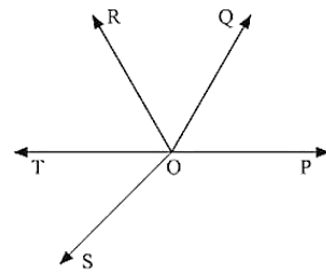Solution:

Given that

OP, OQ, OR and OS are four rays

You need to produce any of the ray OP, OQ, OR and OS backwards to a point in the figure.

Let us produce ray OQ backwards to a point T

So that TOQ is a line

Ray OP stands on the TOQ

Since ∠TOP, ∠POQ is a linear pair

∠TOP + ∠POQ = 180° ... (1)

Similarly,

Ray OS stands on the line TOQ

∠TOS + ∠SOQ = 180° ... (2)

But ∠SOQ = ∠SOR + ∠QOR  ... (3)

So, eqn (2) becomes

∠TOS + ∠SOR + ∠OQR = 180°

Now, adding (1) and (3) you get ∠TOP + ∠POQ + ∠TOS + ∠SOR + ∠QOR = 360° ... (4)

∠TOP + ∠TOS = ∠POS

Eqn: (4) becomes

∠POQ + ∠QOR + ∠SOR + ∠POS = 360°

Question: 17

In below fig, ray OS stand on a line POQ. Ray OR and ray OT are angle bisectors of ∠POS and ∠SOQ respectively. If ∠POS = x, find ∠ROT?Solution:

Given,

Ray OS stand on a line POQ

Ray OR and Ray OT are angle bisectors of ∠POS and ∠SOQ respectively

∠POS = x

∠POS and ∠SOQ is linear pair

∠POS + ∠QOS = 180°

x + QOS = 180

QOS = 180 - x

Now, ray or bisector POS

∠ROS = 1/2 ∠POS

x/2

ROS = x/2    [Since POS = x]

Similarly ray OT bisector QOS

∠TOS = 1/2 ∠QOS

= (180 - x)/2    [QOS = 180 - x]

= 90 - x/2

Hence, ∠ROT = ∠ROS + ∠ROT

= x/2 + 90 - x/2

= 90

∠ROT = 180°

Question: 18

In the below fig, lines PQ and RS intersect each other at point O. If ∠POR: ∠ROQ = 5: 7. Find all the angles.Solution:

Given

∠POR and ∠ROP is linear pair

∠POR + ∠ROP = 180°

Given that

∠POR: ∠ROQ = 5:7

Hence, POR = (5/12) × 180 = 75

Similarly ROQ = (7/7 + 5) × 180 = 105

Now POS = ROQ = 105°  [Vertically opposite angles]

Also, SOQ = POR = 75°   [Vertically opposite angles]

Question: 19

In the below fig. POQ is a line. Ray OR is perpendicular to line PQ. OS is another ray lying between rays OP and OR. Prove that ∠ROS = 1/2(∠QOS − ∠POS).Solution:

Given that

OR perpendicular

∴ ∠POR = 90°

∠POS + ∠SOR = 90         [∴ ∠POR = ∠POS + ∠SOR]

∠ROS = 90° − ∠POS  ... (1)

∠QOR = 90 (∵ OR ⊥ PQ)

∠QOS − ∠ROS = 90°

∠ROS = ∠QOS − 90°

By adding (1) and (2) equations, we get

∴ ∠ROS = ∠QOS − ∠POS

∠ROS = 1/2(∠QOS − ∠POS)
```### Course Features

• 728 Video Lectures
• Revision Notes
• Previous Year Papers
• Mind Map
• Study Planner
• NCERT Solutions
• Discussion Forum
• Test paper with Video Solution